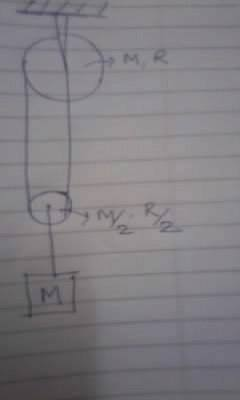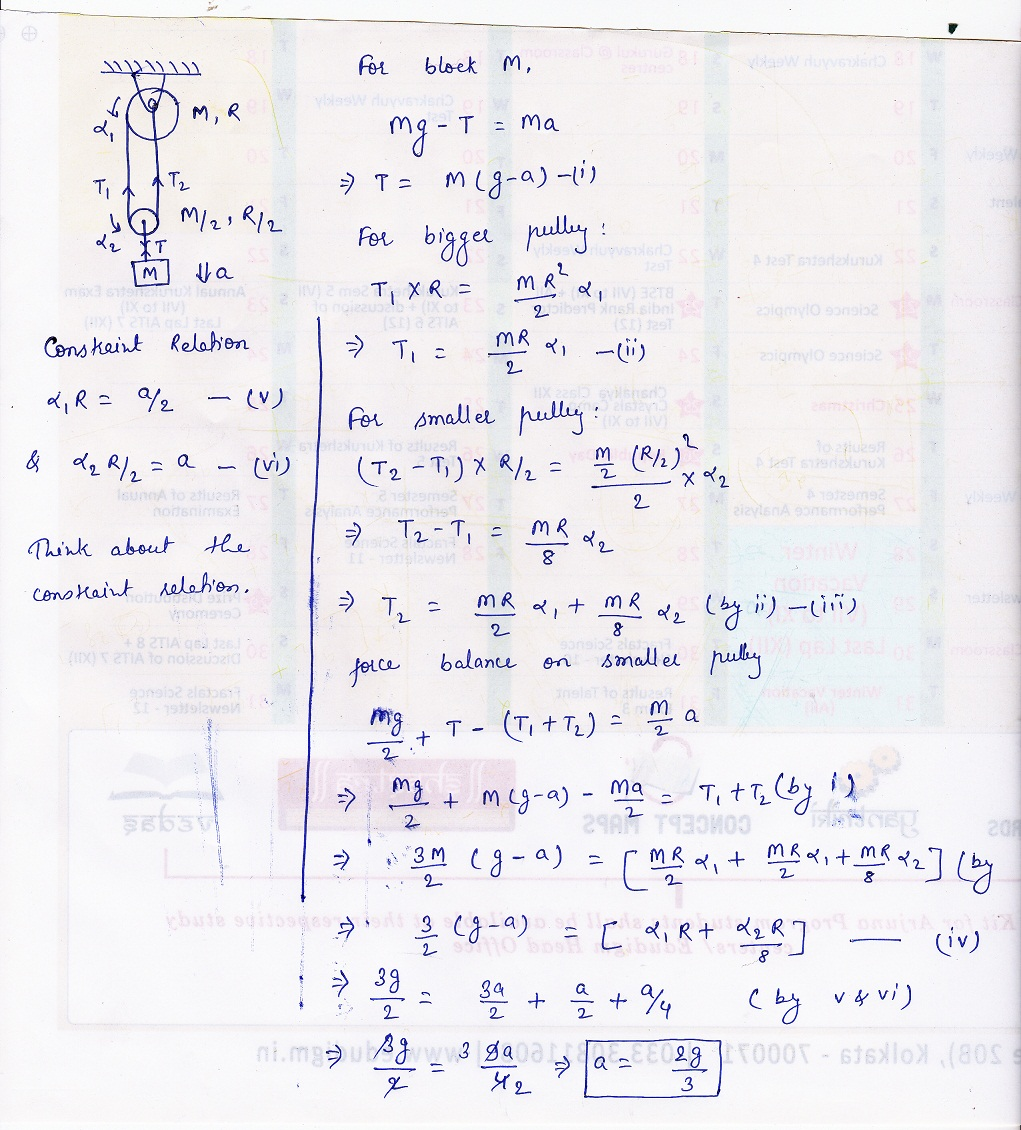# Rotational

A cylindrical pulley of mass M and radius R is connected to another pulley of mass M/2 ,R/2 through a massless string as shown. If sufficient string is wrapped over larger pulley,find the accleration of mass M attached to the smaller pulley when whole system is released from rest .(assume there is no slipping in the smaller pulley)•Sourish Ghosh ·

2g5

Nandikesh Singh ·

Sir,for the first constraint velocity of the string near the bigger pulley and near the smaller pulley must be same.
therefore,
Î±1R=Î±2R/2 + a
and the second one is Î±2R/2=a

solving these two equations
Î±1R=2a and Î±2R=2a.

where am i going wrong?

BabyStealer ·

the correct answer is 2g/5. In AAKASH ANAND'S solution the first constraint relation is wrong. Instead of \alpha_1R=\frac{a}{2}, it must be \alpha_1R=2a. Rest of the equations are correct. This change will give the correct answer. that is \frac{2g}{5}.

•Akash Anand ·•Sourish Ghosh I gave this a lot of thought but still can't figure out how to derive the relation. It is clear that the smaller pulley will rotate quicker than the larger one. But what concept to use to find the relation? Do we have to use the "net work done = 0" concept? If so, can you show how?
•Akash Anand If you think a bit like..rotation of smaller pulley won't make any difference for length of string. If smaller pulley would be massless then also the constraint relation will be as in this case, because putting mass on the lower pulley is not going to contribute in any way. For the second constraint relation, I am sure you don't have any doubt.
•Anonymous Can you please explain how has the constraint been derived? And the answer given 2g/5
•